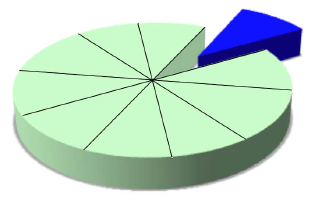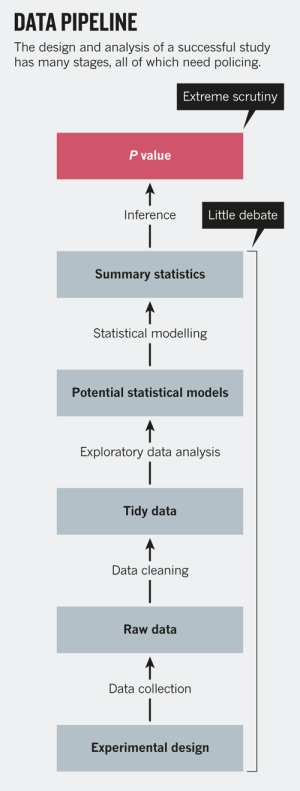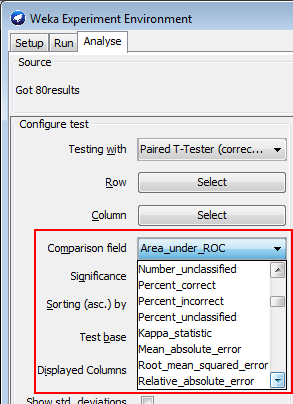# Statistics - Model Evaluation (Estimation|Validation|Testing)

Evaluation is how to determine if the model is a good representation of the truth.

Validation applies the model to test data in order to determine whether the model, built on a training set, is generalizable to other data. In particular, it helps to avoid the phenomenon of overfitting, which can occur when the logic of the model fits the build data too well and therefore has little predictive power.

Validation is a process that help to tell how well does a model in terms of test error

• the training set for building the model
• the test set use in this validation step in order to evaluate the model

However, if the data is already split into Build and Test subsets, you can specify them.

• Beware of the human tendency to see patterns in random data. See wiki/Apophenia

## Metrics

• Classifier =
• % of correct predictions
• Regression = Error calculation
• Evaluate the error from a test set where every rating is the average rating for the training set.

## Documentation / Reference

Discover More(Machine|Statistical) Learning - (Target|Learned|Outcome|Dependent|Response) (Attribute|Variable) (Y|DV)

An (outcome|dependent) variable is ameasure that we want to predict. : the original score collected : the predicted score (or estimator) from the equation. The hat means “estimated” from the...(Statistics|Data Mining) - (K-Fold) Cross-validation (rotation estimation)

Cross-validation, sometimes called rotation estimation is a resampling validation technique for assessing how the results of a statistical analysis will generalize to an independent new data set. This...Data Mining - (Function|Model)

The model is the function, equation, algorithm that predicts an outcome value from one of several predictors. During the training process, the models are build. A model uses a logic and one of several...Data Mining - (Life cycle|Project|Data Pipeline)

Data mining is an experimental science. Data mining reveals correlation, not causation. With good data, you will make good algorithm. The most preferable solution is then to work on good features....Data Mining - (Parameters | Model) (Accuracy | Precision | Fit | Performance) Metrics

Accuracy is a evaluation metrics on how a model perform. rare event detection Hypothesis testing: t-statistic and p-value. The p value and t statistic measure how strong is the...Data Mining - Data (Preparation | Wrangling | Munging)

Data for mining must exist within a single table or view. The information for each case (record) must be stored in a separate row. Proper preparation of the data is a key factor in any data mining project....Data Mining - Test Set

The test set is a set that is used to validate the model. Test set represent the foresight (unknown data, real data) whereas training Set represents the hindsight. Generally, the test data is created...Loss functions (Incorrect predictions penalty)

Loss functions define how to penalize incorrect predictions. The optimization problems associated with various linear classifiers are defined as minimizing the loss on training points (sometime along with...Machine Learning - (Overfitting|Overtraining|Robust|Generalization) (Underfitting)

A learning algorithm is said to overfit if it is: more accurate in fitting known data (ie training data) (hindsight) but less accurate in predicting new data (ie test data) (foresight) Ie the model...Machine Learning - (Supervised|Directed) Learning ( Training ) (Problem)

Supervised Learning has the goal of predicting a value (outcome) from particular characteristics (predictors) that describes some behaviour. The attribute used to trained and being predicted is called...